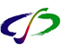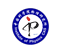引用本文: 彭芳麟. “图解”数学物理方法的教学实践[J]. 物理, 2007, 36(02): 153-158.Multimedia applying in course of methods of mathematical physics[J]. PHYSICS, 2007, 36(02): 153-158.
 Citation: Multimedia applying in course of methods of mathematical physics[J]. PHYSICS, 2007, 36(02): 153-158.## Multimedia applying in course of methods of mathematical physics

• 摘要: 《数学物理方法》虽然是数学课，但是它是介绍在物理中应用的数学.物理课程多媒体教学的经验启发我们，合理地选择《数学物理方法》课程中有物理背景的内容，恰当地使用可视化以展现数学公式的物理图像，就能提高教学效果,对一些随时间演变的方程,还可以用动画来表现.我们用两种方法实现可视化，一是用解析解作图，另一个是通过数值求解方程,再将数值计算结果作图.本文介绍我们在《数学物理方法》课开展可视化教学的实践.

Abstract: “Methods of mathematical physics” is a course which introduces the mathematics applied in physics. The experience from multimedia instruction in physics suggests that by appropriately selecting the content related to physics and the visualization that exhibits the physical picture of the mathematical formulae (including the use of movie to demonstrate time dependent processes)., the effectiveness of the instruction may be improved. In our course, we have adopted two methods of visualization: one is to plot directly the analytical solution, the other is to first solve the equations numerically and then plot the resulting numerical solutions. Our practice and experience are presented in this article./下载:  全尺寸图片 幻灯片
• 分享
• 用微信扫码二维码

分享至好友和朋友圈